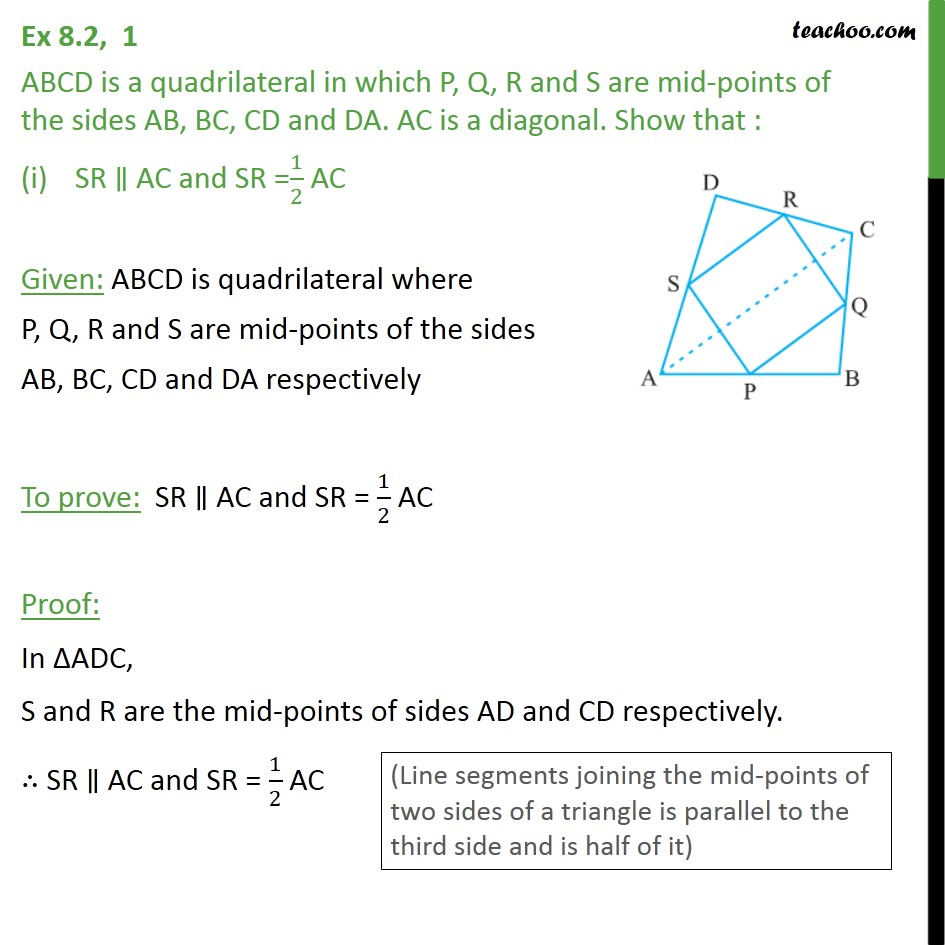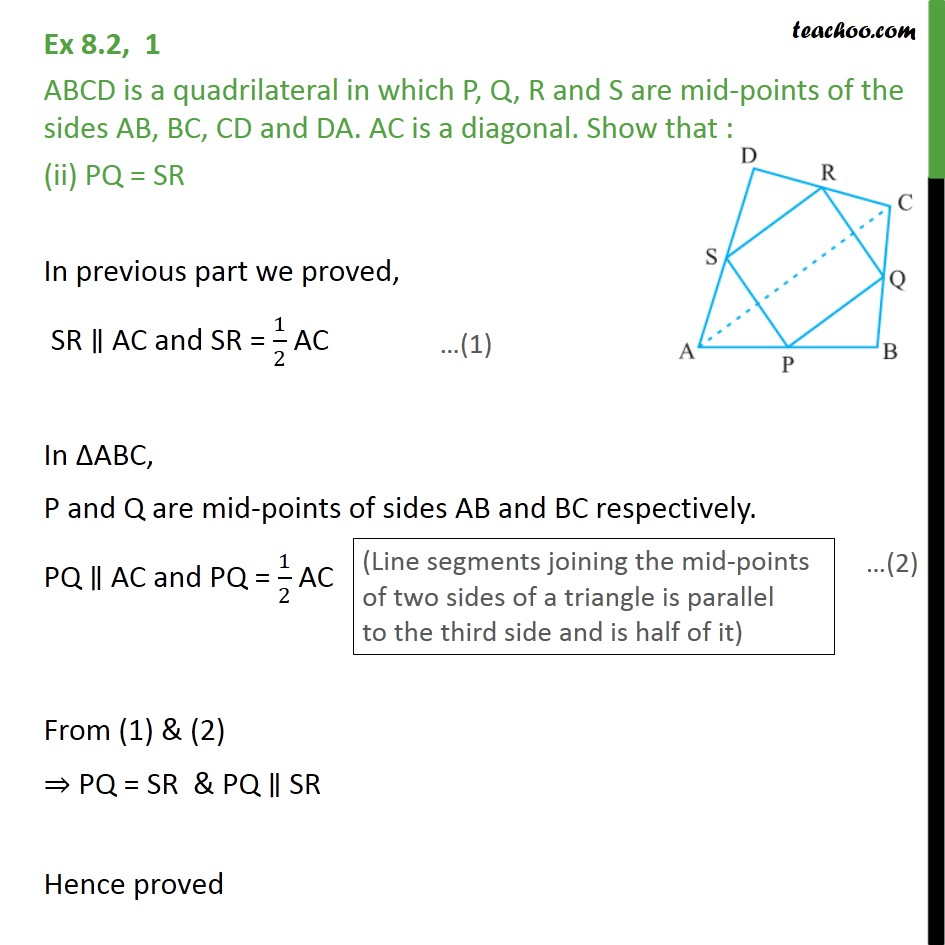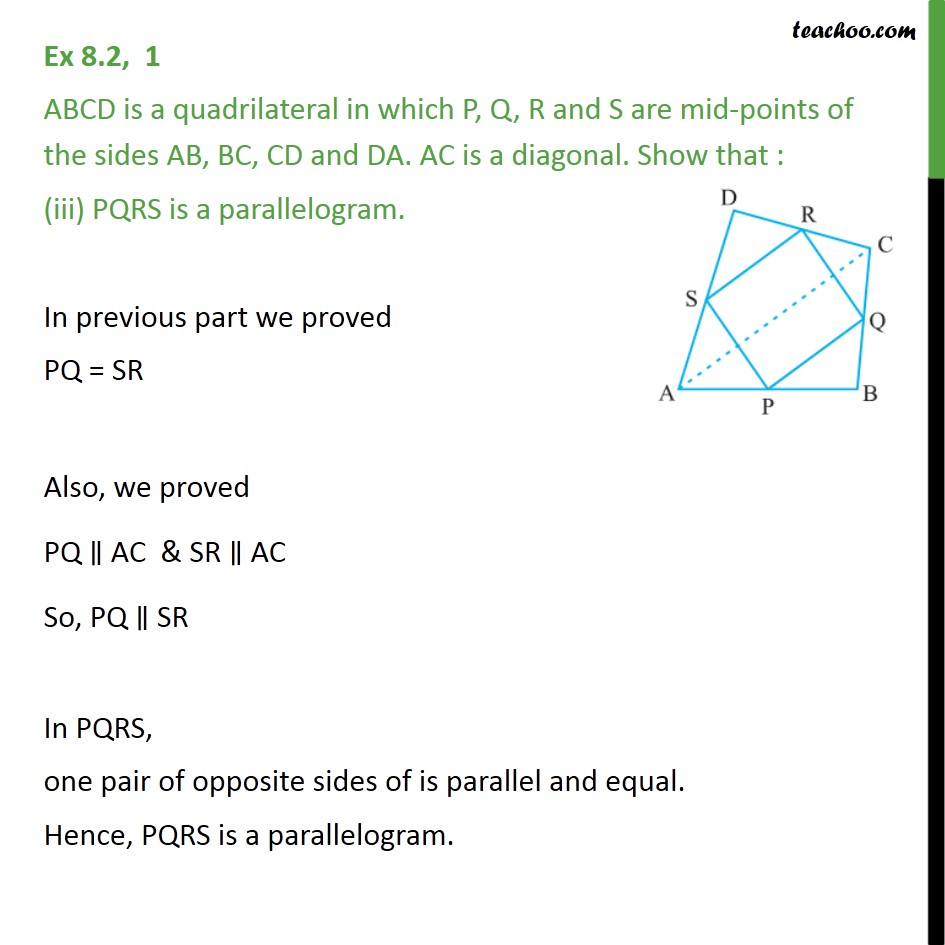1. Chapter 8 Class 9 Quadrilaterals (Term 2)
2. Serial order wise
3. Ex 8.2

Transcript

Ex 8.2, 1 ABCD is a quadrilateral in which P, Q, R and S are mid-points of the sides AB, BC, CD and DA. AC is a diagonal. Show that : SR ∥ AC and SR =1/2 AC Given: ABCD is quadrilateral where P, Q, R and S are mid-points of the sides AB, BC, CD and DA respectively To prove: SR ∥ AC and SR = 1/2 AC Proof: In ΔADC, S and R are the mid-points of sides AD and CD respectively. ∴ SR ∥ AC and SR = 1/2 AC Ex 8.2, 1 ABCD is a quadrilateral in which P, Q, R and S are mid-points of the sides AB, BC, CD and DA. AC is a diagonal. Show that : (ii) PQ = SR In previous part we proved, SR ∥ AC and SR = 1/2 AC In ΔABC, P and Q are mid-points of sides AB and BC respectively. PQ ∥ AC and PQ = 1/2 AC From (1) & (2) ⇒ PQ = SR & PQ ∥ SR Hence proved Ex 8.2, 1 ABCD is a quadrilateral in which P, Q, R and S are mid-points of the sides AB, BC, CD and DA. AC is a diagonal. Show that : (iii) PQRS is a parallelogram. In previous part we proved PQ = SR Also, we proved PQ ∥ AC & SR ∥ AC So, PQ ∥ SR In PQRS, one pair of opposite sides of is parallel and equal. Hence, PQRS is a parallelogram.